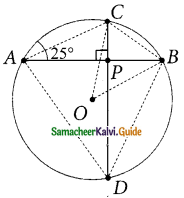Students can download Maths Chapter 4 Geometry Ex 4.3 Questions and Answers, Notes, Samacheer Kalvi 9th Maths Guide Pdf helps you to revise the complete Tamilnadu State Board New Syllabus, helps students complete homework assignments and to score high marks in board exams.

## Tamilnadu Samacheer Kalvi 9th Maths Solutions Chapter 4 Geometry Ex 4.3

Question 1.
The diameter of the circle is 52 cm and the length of one of its chord is 20 cm. Find the distance of the chord from the centre.
Radius of a circle = $$\frac{56}{2}$$ = 26 cm
Solution: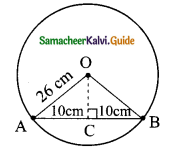Length of the chord = 20 cm
AC = $$\frac{20}{2}$$
= 10 cm
In ΔOAC, OC² = OA² – AC²
= 26² – 10²
= (26 + 10) (26 – 10)
= 36 × 16
OC = $$\sqrt{30×16}$$
= 6 × 4 cm
= 24 cm
Distance of the chord from the centre = 24 cm.Question 2.
The chord of length 30 cm is drawn at the distance of 8 cm from the centre of the circle. Find the radius of the circle.
Solution: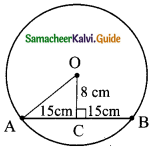Distance AC = $$\frac{1}{2}$$ × Length of chord
= $$\frac{1}{2}$$ × 30
= 15 cm
Distance from the centre = 8 cm
In ΔOAC Radius (OA) = $$\sqrt{AC² + OC²}$$
= $$\sqrt{15² + 8²}$$
= $$\sqrt{224 + 64}$$
= $$\sqrt{289}$$
= 17
Radius of the circle = 17 cm.Question 3.
Find the length of the chord AC where AB and CD are the two diameters perpendicular to each other of a circle with radius 4√2 cm and also find ∠OAC and ∠OCA.
Solution:
Radius of a circle = 4√2 cm
In the right ΔAOC,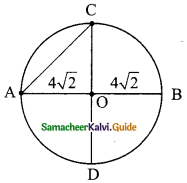AC² = OA² + OC²
AC² = (4√2)² + (4√2)²
= 32 + 32 = 64
AC = $$\sqrt{64}$$
= 8
Length of the chord = 8 cm,
∠OAC = ∠OCA = 45°
Since OAC is an isosceles right angle triangle.Question 4.
A chord is 12 cm away from the centre of the circle of radius 15cm. Find the length of the chord.
Solution:
Radius of a circle (OA) = 15 cm
Distance from centre to the chord (OC) = 12 cm
In the right ΔOAC,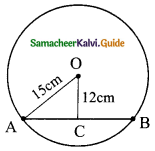AC² = OA² – OC²
= 15² – 12²
= 225 – 144
= 81
AC = $$\sqrt{81}$$
= 9
Length of the chord (AB) = AC + CB
= 9 + 9 = 18 cm.Question 5.
In a circle, AB and CD are two parallel chords with centre O and radius 10 cm such that AB = 16 cm and CD = 12 cm determine the distance between the two chords?
Solution:
Length of the chord (AB) = 16 cm
∴ AF = $$\frac{1}{2}$$ × 16
= 8 cm
Length of the chord (CD) = 12 cm
∴ CE = $$\frac{1}{2}$$ × 12
= 6 cm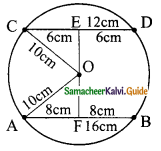In the right ΔOCE,
OE² = OC² – CE²
= 10² – 6²
= 100 – 36
= 64
OE = $$\sqrt{64}$$
= 8 cm
In the right ΔOAF,
OF² = OA² – AF²
= 10² – 8²
= 100 – 64
= 36
OE = $$\sqrt{36}$$
= 6 cm
Distance between the two chords = OE + OF
= 8 + 6
= 14 cm.Question 6.
Two circles of radii 5 cm and 3 cm intersect at two points and the distance between their centres is 4 cm. Find the length of the common chord.
Ans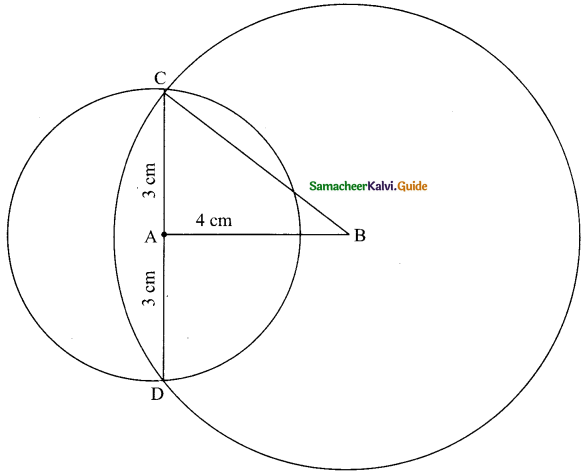Two circules intersect at C and D
CD is the common chord.
= 3 + 3
= 6 cm
Length of the common chord = 6 cm.Question 7.
Find the value of x° in the following figures: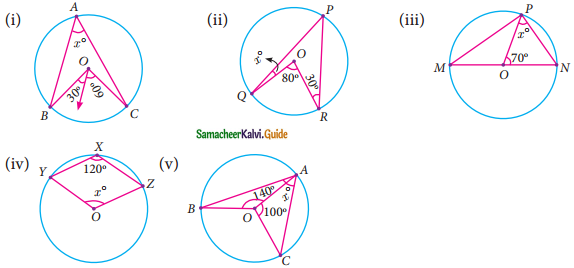Solution:
(i) ∠BOC = 30° + 60°
= 90°
∠BAC (x) = $$\frac{1}{2}$$ ∠BOC (By theorem)
= $$\frac{1}{2}$$ × 90°
x = 45°

(ii) Join OP
∠QPR = $$\frac{80}{2}$$ = 40° (Angle of the circumference is $$\frac{1}{2}$$ the angle at the centre)
∠RPO = 30° (OP and OR are equal sides)
∠OPQ = 40° – 30°
= 10°
∠OQP = 10° (OQ and OP are equal sides)
∴ x = 10°(iii) ∠OPN = ∠ONP (ON and OP are the radius of the circle)
= 90°
In ΔOPN
∠PON + ∠ONP + ∠NPO = 180° (Sum of the angles of a triangle)
70° + x° + x° = 180°
2x° + 70° = 180°
2x = 180° – 70°
2x = 110°
X = $$\frac{110°}{2}$$
= 55°
The value of x = 55°

(iv) Reflex ∠YOZ = 2∠YXZ
= 2(120°)
= 240°
∠YOZ = 360° – reflex ∠YOZ
= 360° – 240°
= 120°
∴ x = 120°

(v) ∠OAC + ∠OCA = 180° – 100°
= 80°
∠OAC = $$\frac{1}{2}$$ × 80° [Since OA = OC, (∴ ∠OAC = ∠OCA)]
= 40°
∠OBA + ∠OAB = 180° – 140°
= 40° [Since ∠OBA = ∠OAB, since OB = OA]
∴∠OAB = $$\frac{40°}{2}$$
= 20°
∠BAC = ∠OAB + ∠OAC
= 20° + 40°
x = 60°Question 8.
In the given figure, ∠CAB = 25°, find ∠BDC, ∠DBA and ∠COB.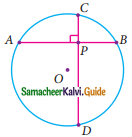Solution:
In ΔACP,
∠ACP = 180° – (25° + 90°)
= 180° – 115°
= 65°
∠CBA = ∠CAB = 25° [Both the angles are standing in the same base]
∠DBA = 65° [∠DBA and ∠BCA standing in the same base]
∠COB = 50°